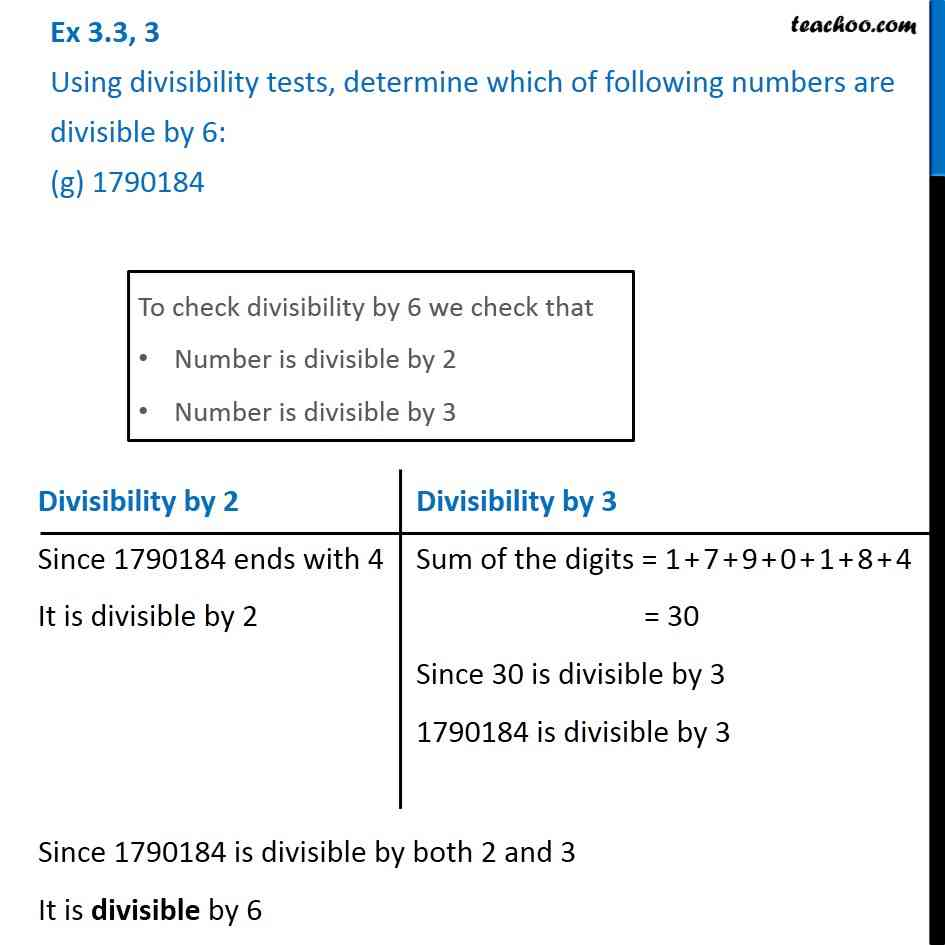Ex 3.3

Chapter 3 Class 6 Playing with Numbers
Serial order wiseLearn in your speed, with individual attention - Teachoo Maths 1-on-1 Class

### Transcript

Ex 3.3, 3 Using divisibility tests, determine which of following numbers are divisible by 6: (g) 1790184To check divisibility by 6 we check that Number is divisible by 2 Number is divisible by 3 Divisibility by 2 Since 1790184 ends with 4 It is divisible by 2 Divisibility by 3 Sum of the digits = 1 + 7 + 9 + 0 + 1 + 8 + 4 = 30 Since 30 is divisible by 3 1790184 is divisible by 3 Since 1790184 is divisible by both 2 and 3 It is divisible by 6